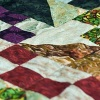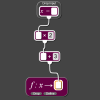# Resources tagged with: Factors and multiples

Filter by: Content type:
Age range:
Challenge level:

### There are 157 results

Broad Topics > Properties of Numbers > Factors and multiples### Three Times Seven

##### Age 11 to 14Challenge Level

A three digit number abc is always divisible by 7 when 2a+3b+c is divisible by 7. Why?### Counting Factors

##### Age 11 to 14Challenge Level

Is there an efficient way to work out how many factors a large number has?### What Numbers Can We Make Now?

##### Age 11 to 14Challenge Level

Imagine we have four bags containing numbers from a sequence. What numbers can we make now?##### Age 11 to 14Challenge Level

List any 3 numbers. It is always possible to find a subset of adjacent numbers that add up to a multiple of 3. Can you explain why and prove it?### Repeaters

##### Age 11 to 14Challenge Level

Choose any 3 digits and make a 6 digit number by repeating the 3 digits in the same order (e.g. 594594). Explain why whatever digits you choose the number will always be divisible by 7, 11 and 13.### Hot Pursuit

##### Age 11 to 14Challenge Level

I added together the first 'n' positive integers and found that my answer was a 3 digit number in which all the digits were the same...### Even So

##### Age 11 to 14Challenge Level

Find some triples of whole numbers a, b and c such that a^2 + b^2 + c^2 is a multiple of 4. Is it necessarily the case that a, b and c must all be even? If so, can you explain why?### Special Sums and Products

##### Age 11 to 14Challenge Level

Find some examples of pairs of numbers such that their sum is a factor of their product. eg. 4 + 12 = 16 and 4 × 12 = 48 and 16 is a factor of 48.### Can You Find a Perfect Number?

##### Age 7 to 14### Take Three from Five

##### Age 11 to 16Challenge Level

Caroline and James pick sets of five numbers. Charlie chooses three of them that add together to make a multiple of three. Can they stop him?##### Age 11 to 14Challenge Level

Make a set of numbers that use all the digits from 1 to 9, once and once only. Add them up. The result is divisible by 9. Add each of the digits in the new number. What is their sum? Now try some. . . .### Just Repeat

##### Age 11 to 14Challenge Level

Think of any three-digit number. Repeat the digits. The 6-digit number that you end up with is divisible by 91. Is this a coincidence?### Big Powers

##### Age 11 to 16Challenge Level

Three people chose this as a favourite problem. It is the sort of problem that needs thinking time - but once the connection is made it gives access to many similar ideas.### Inclusion Exclusion

##### Age 11 to 14Challenge Level

How many integers between 1 and 1200 are NOT multiples of any of the numbers 2, 3 or 5?### Alison's Quilt

##### Age 11 to 14Challenge Level

Nine squares are fitted together to form a rectangle. Can you find its dimensions?### Got It

##### Age 7 to 14Challenge Level

A game for two people, or play online. Given a target number, say 23, and a range of numbers to choose from, say 1-4, players take it in turns to add to the running total to hit their target.### Remainder

##### Age 11 to 14Challenge Level

What is the remainder when 2^2002 is divided by 7? What happens with different powers of 2?### Shifting Times Tables

##### Age 11 to 14Challenge Level

Can you find a way to identify times tables after they have been shifted up or down?### Counting Cogs

##### Age 7 to 14Challenge Level

Which pairs of cogs let the coloured tooth touch every tooth on the other cog? Which pairs do not let this happen? Why?### Exploring Simple Mappings

##### Age 11 to 14Challenge Level

Explore the relationship between simple linear functions and their graphs.### What Numbers Can We Make?

##### Age 11 to 14Challenge Level

Imagine we have four bags containing a large number of 1s, 4s, 7s and 10s. What numbers can we make?### Factor Track

##### Age 7 to 14Challenge Level

Factor track is not a race but a game of skill. The idea is to go round the track in as few moves as possible, keeping to the rules.### Number Families

##### Age 11 to 14Challenge Level

How many different number families can you find?### Got it for Two

##### Age 7 to 14Challenge Level

Got It game for an adult and child. How can you play so that you know you will always win?### Gabriel's Problem

##### Age 11 to 14Challenge Level

Gabriel multiplied together some numbers and then erased them. Can you figure out where each number was?### Satisfying Statements

##### Age 11 to 14Challenge Level

Can you find any two-digit numbers that satisfy all of these statements?### Divisibility Tests

##### Age 11 to 16

This article explains various divisibility rules and why they work. An article to read with pencil and paper handy.##### Age 7 to 14Challenge Level

I added together some of my neighbours' house numbers. Can you explain the patterns I noticed?### Funny Factorisation

##### Age 11 to 16Challenge Level

Using the digits 1 to 9, the number 4396 can be written as the product of two numbers. Can you find the factors?### Summing Consecutive Numbers

##### Age 11 to 14Challenge Level

15 = 7 + 8 and 10 = 1 + 2 + 3 + 4. Can you say which numbers can be expressed as the sum of two or more consecutive integers?### Dozens

##### Age 7 to 14Challenge Level

Can you select the missing digit(s) to find the largest multiple?### Cogs

##### Age 11 to 14Challenge Level

A and B are two interlocking cogwheels having p teeth and q teeth respectively. One tooth on B is painted red. Find the values of p and q for which the red tooth on B contacts every gap on the. . . .### Have You Got It?

##### Age 11 to 14Challenge Level

Can you explain the strategy for winning this game with any target?##### Age 11 to 14Challenge Level

Great Granddad is very proud of his telegram from the Queen congratulating him on his hundredth birthday and he has friends who are even older than he is... When was he born?### Two Much

##### Age 11 to 14Challenge Level

Explain why the arithmetic sequence 1, 14, 27, 40, ... contains many terms of the form 222...2 where only the digit 2 appears.### Eminit

##### Age 11 to 14Challenge Level

The number 8888...88M9999...99 is divisible by 7 and it starts with the digit 8 repeated 50 times and ends with the digit 9 repeated 50 times. What is the value of the digit M?### Thirty Six Exactly

##### Age 11 to 14Challenge Level

The number 12 = 2^2 × 3 has 6 factors. What is the smallest natural number with exactly 36 factors?### American Billions

##### Age 11 to 14Challenge Level

Play the divisibility game to create numbers in which the first two digits make a number divisible by 2, the first three digits make a number divisible by 3...### Gaxinta

##### Age 11 to 14Challenge Level

A number N is divisible by 10, 90, 98 and 882 but it is NOT divisible by 50 or 270 or 686 or 1764. It is also known that N is a factor of 9261000. What is N?### Making Pathways

##### Age 7 to 11Challenge Level

Can you find different ways of creating paths using these paving slabs?### How Old Are the Children?

##### Age 11 to 14Challenge Level

A student in a maths class was trying to get some information from her teacher. She was given some clues and then the teacher ended by saying, "Well, how old are they?"### Flow Chart

##### Age 11 to 14Challenge Level

The flow chart requires two numbers, M and N. Select several values for M and try to establish what the flow chart does.### X Marks the Spot

##### Age 11 to 14Challenge Level

When the number x 1 x x x is multiplied by 417 this gives the answer 9 x x x 0 5 7. Find the missing digits, each of which is represented by an "x" .### Factoring Factorials

##### Age 11 to 14Challenge Level

Find the highest power of 11 that will divide into 1000! exactly.### Multiplication Series: Number Arrays

##### Age 5 to 11

This article for teachers describes how number arrays can be a useful representation for many number concepts.### Cuboids

##### Age 11 to 14Challenge Level

Can you find a cuboid that has a surface area of exactly 100 square units. Is there more than one? Can you find them all?### Crossings

##### Age 7 to 11Challenge Level

In this problem we are looking at sets of parallel sticks that cross each other. What is the least number of crossings you can make? And the greatest?### Product Sudoku

##### Age 11 to 16Challenge Level

The clues for this Sudoku are the product of the numbers in adjacent squares.### Star Product Sudoku

##### Age 11 to 16Challenge Level

The puzzle can be solved by finding the values of the unknown digits (all indicated by asterisks) in the squares of the $9\times9$ grid.### Divisively So

##### Age 11 to 14Challenge Level

How many numbers less than 1000 are NOT divisible by either: a) 2 or 5; or b) 2, 5 or 7?